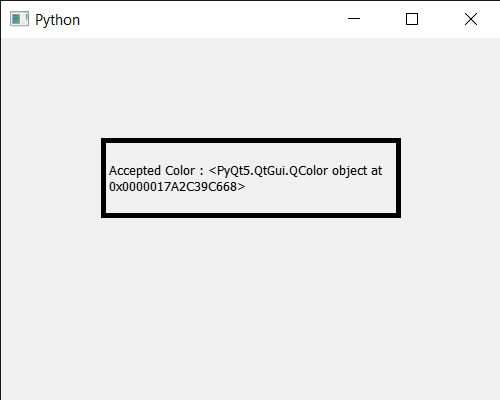GeeksforGeeks App
Open AppBrowser
Continue

# PyQt5 QColorDialog – Rejected Signal

In this article we will see how we can get the rejected signal of the QColorDialog widget. This signal is emitted when the dialog has been rejected either by the user or by calling `reject()` or `done()` with the QDialog::Rejected argument.

Note : This signal is not emitted when hiding the dialog with hide() or setVisible(false). This includes deleting the dialog while it is visible.

In order to do this we use `rejected` method with the QColorDialog object

Syntax : dialog.rejected.connect(lambda: print(“Rejected Signal”)

Argument : It takes method as argument

Action Performed : It will call the passed method when rejected signal emitted

Below is the implementation

 `# importing libraries``from` `PyQt5.QtWidgets ``import` `*` `from` `PyQt5 ``import` `QtCore, QtGui``from` `PyQt5.QtGui ``import` `*` `from` `PyQt5.QtCore ``import` `*` `import` `sys`` ` ` ` `class` `Window(QMainWindow):`` ` `    ``def` `__init__(``self``):``        ``super``().__init__()`` ` `        ``# setting title``        ``self``.setWindowTitle(``"Python "``)`` ` `        ``# setting geometry``        ``self``.setGeometry(``100``, ``100``, ``500``, ``400``)`` ` `        ``# calling method``        ``self``.UiComponents()`` ` `        ``# showing all the widgets``        ``self``.show()`` ` ` ` `    ``# method for components``    ``def` `UiComponents(``self``):`` ` `        ``# creating a QColorDialog object``        ``dialog ``=` `QColorDialog(``self``)`` ` `        ``# setting current color to the dialog``        ``dialog.setCurrentColor(Qt.red)`` ` `        ``# getting rejected signal``        ``dialog.rejected.connect(``lambda``: ``print``(``"Rejected  Signal"``))`` ` `        ``# making color done``        ``# rejecting the color``        ``dialog.done(``0``)`` ` `        ``# executing the dialog``        ``dialog.exec_()`` ` ` ` `        ``# creating label``        ``label ``=` `QLabel(``"GeeksforGeeks"``, ``self``)`` ` `        ``# setting geometry to the label``        ``label.setGeometry(``100``, ``100``, ``300``, ``80``)`` ` `        ``# making label multi line``        ``label.setWordWrap(``True``)`` ` `        ``# setting stylesheet of the label``        ``label.setStyleSheet(``"QLabel"``                            ``"{"``                            ``"border : 5px solid black;"``                            ``"}"``)`` ` `        ``# getting the selected color``        ``color ``=` `dialog.selectedColor()`` ` `        ``# setting graphic effect to the label``        ``graphic ``=` `QGraphicsColorizeEffect(``self``)`` ` `        ``# setting color to the graphic``        ``graphic.setColor(color)`` ` `        ``# setting graphic to the label``        ``label.setGraphicsEffect(graphic)`` ` `        ``# setting text to the label``        ``label.setText(``"Accepted Color : "` `+` `str``(color))`` ` ` ` ` ` ` ` `# create pyqt5 app``App ``=` `QApplication(sys.argv)`` ` `# create the instance of our Window``window ``=` `Window()`` ` `# start the app``sys.exit(App.``exec``())`

Output :

```Rejected  Signal
Rejected  Signal
```My Personal Notes arrow_drop_up